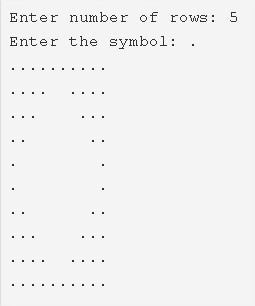# C Program Hollow Diamond Star Pattern | C Programs

C Program to print hollow diamond star pattern – In this article, we will detail in on the several ways to print a hollow diamond star pattern in C programming.

Suitable examples and sample programs have also been added so that you can understand the whole thing very clearly. The compiler has also been added with which you can execute it yourself.

The methods used to do so are as follows:

• Using For Loop
• Using While Loop
• Using Do-While Loop

As the title of the piece suggests, you have to print a hollow diamond star pattern.

It looks like this:As you can see, you need to enter the number of rows first up. Then, it will print the hollow diamond star pattern with the number of rows from the centre towards the top and the bottom.

Here are number of ways for Hollow Diamond Star Pattern Program in C:

## Using For Loop

1. Read the rows number which is entered by the user and store the value into the variable n. Read the entered symbol and store the symbol into the variable ch.

2) The 1st for loop iterates through rows with the structure for(i=0;i<n;i++).

a) The 2nd for loop iterates through columns with the structure for(j=0;j<n;j++).

a.1) If j<n-i condition is true then it prints symbol.

a.2) If the condition j<n-i is false then it prints space.

b) The 3rd for loop iterates through columns with the structure for(j=0;j<n;j++).

b.1) If j<i condition is true then it prints space.

b.2) If the condition j<i is false then it prints symbol.

c) The cursor comes to next line for each iteration of the 1st for the loop.

After the above steps, we will get the half part of the hollow diamond star pattern.

3) The 4th for loop iterates through rows with the structure for(i=1;i<=n;i++).

a) The 5th for loop iterates through columns with the structure for(j=0;j<n;j++).

a.1) If j<i condition is true then it prints symbol.

a.2) If the condition j<i is false then it prints space.

b) The 5th for loop iterates through columns with the structure for(j=0;j<n;j++).

b.1) If the condition j<n-i is true then it prints space.

b.2) If the condition j<n-i is false then it prints symbol.

c) The cursor comes to next line for each iteration of outer for loop.

After the above steps, we will get the remaining half part of the hollow diamond star pattern.

Output:

### Using While Loop

1. i=0; The 1st while loop with the condition i<n iterates through rows until the condition becomes false.

a) j=0, The 2nd while loop with the condition j<n iterates through columns until the condition becomes false.

a.1) if(j<n-i) condition is true then it prints symbol.Otherwise, it prints space.

a.2) j value increased by 1.

b) j=0, The 3rd while loop iterates through columns until the condition j<n becomes false.

b.1) if(j<i) condition is true then it prints space.Otherwise, it prints symbol.

b.2) j value increased by 1.

c) Cursor comes to the next line for each iteration of the outer while loop.i value increased by 1.

From the above steps, we will get the half part of the hollow diamond star pattern.

2) i=1, The 4th while loop iterates through rows until the condition i<=n becomes false.

a) j=0, the 5th while loop iterates through columns until the condition j<n becomes false.

a.1) If the condition j<i is true then it prints symbol. Otherwise, it prints space.

a.2) j value increased by 1.

b) j=0, the 6th while loop iterates through columns until the condition j<n becomes false.

b.1) If the condition j<n-i is true then it prints space. Otherwise, it prints symbol.

b.2) j value increased by 1.

3) Cursor comes to next line.i value increased by 1.

From the above steps, we will get the remaining half part of the hollow diamond star pattern.

Output:

#### Using Do-While Loop

1. i=0, The 1st do-while loop iterates through rows.

a) j=0, The 2nd do-while loop iterates through columns.

a.1) It prints symbol if j<n-i condition is true. Otherwise, it prints space.

a.2) j value increased by 1.

a.3) Checks the condition j<n. If the condition is true then 2nd do-while loop iterates again.Repeats until the condition becomes false.

b) j=0, the 3rd do-while loop iterates through columns.

b.1) It prints space if j<i is true. Otherwise, it prints symbol.

b.2) j value increased by 1.

b.3) Checks the condition j<n. If the condition is true then loop iterates again.Repeats until the condition becomes false.

c) The cursor comes to next line. i value increased by 1.Checks the condition i<n. If the condition is true then loop iterates again.Repeats until the condition becomes false.

From the above steps, we will get upper half part of the hollow diamond star pattern.

2) i=1, The 4th do-while loop iterates through rows.

a) j=0, The  5th do-while loop iterates through columns.

a.1) It prints symbol if j<i is true. Otherwise, it prints space.

a.2) j value increased by 1.

a.3) Checks the condition j<n. If the condition is true then 5th loop iterates again.Repeats until the condition becomes false.

b) j=0, the 6’th do-while loop iterates through columns.

b.1) It prints space if j<n-i is true. Otherwise, it prints symbol.

b.2) j value increased by 1.

b.3) Checks the condition j<n.

3) The cursor comes to next line.i value increased by 1.Checks the condition i<=n. If the condition is true

Checks the condition i<=n.If the condition is true then the 4th do-while loop iterates again.Repeats until the condition becomes false.

After the above steps, the remaining half part of the hollow diamond star pattern will be printed.

Output:
x

## C Program To Print Number Of Days In A Month | Java Tutoring

C program to input the month number and print the number of days in that ...